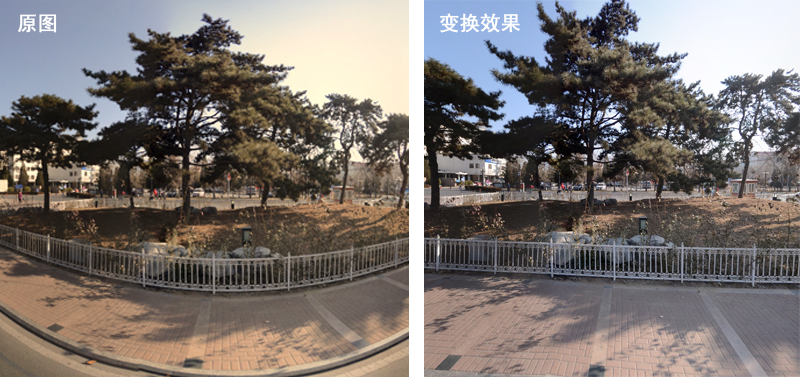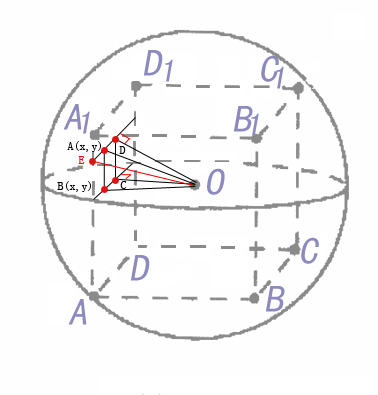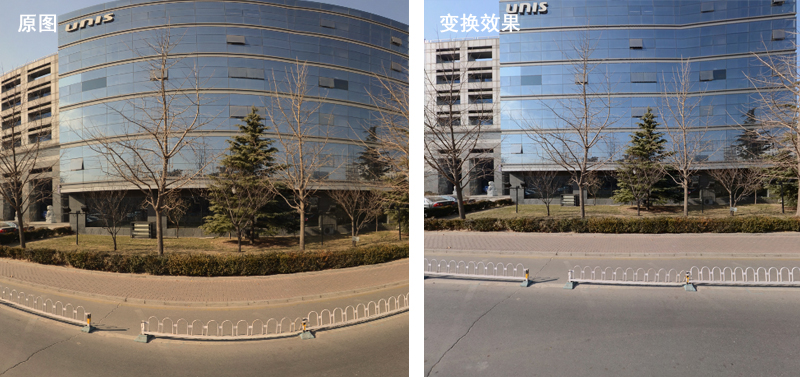鱼眼图片转化位全景图片的软件以及鱼眼矫正方法最近做了一个全景图片平面映射的工作，就是将一个360度的全景球面照片映射到一个平面上，使之看上去没有变形。由于网上的一些鱼眼照片的校正程序不好用，自己通过球体的三角计算，找到了映射效果较好的方法。写博客以备忘。先看看效果。做完以后又进行了一些改造，使程序变成一个可变大小，可变角度的相对较通用的程序。本人能力偏弱，只能用笨办法想问题，不足之处敬请海涵。主要的算法是这样的。当从平面转成球面的时候，需要从圆心引一条直线，通过平面图片的每一个像素，打到球面上，把这个平面的像素给球面即可。我想平面的映射应该就是反过程。关键是怎么求球面的点与平面的点之间的关系。因为虽然是球面的图片，但是只是视觉上是球面的，其图片结构还是二位的像素点。我们来看看具体的分析。

图片上就是我手绘的一个示意图。将球面映射到平面时，需要新建一个平面图片。对平面的图片的横纵左边点循环，跑遍每一个点，做点与圆心o的连线，打到球面上，球面上点与球面图片的左右边界之间的弧长，就是我们要求的球面图片的横坐标。那么弧长如何计算呢？很显然就是使用圆心角的角度乘以球的半径。（因为过圆心，所以是大圆。半径就是把图片的宽度作为大圆周长，求出来的半径R）。圆心角如何求呢？我能想到的比较直接的办法是三角几何。图片上的A点就是我们的平面上任意一点，我们要求的是Ao与红线的夹角。那么就是Do与红线夹角减去Do与Ao夹角就是了。Do与红线夹角为平面图片长度的一般，假设是length/2。Do的长度可以用DCo这个直角三角形，通过勾股定理来求，值为sqrt（y*y+length/2*length/2）。红线与A所在水平直线的交点假设为E，那么ED就是length/2。所以DoE=arctan（ED/Do）。DoA=arctan((length/2-x)/Do)。那么我们求的AoE就是DoE-DoA=arctan（ED/Do）-arctan((length/2-x)/Do)。用这个角度直接乘以半径R就是横坐标了。当点在平面的右半部分时，把length/2-x变成x-length/2即可。

纵坐标的求取跟这个很像，只需要求AoB的角度。通过BC与oC的直角三角形勾股定理求Bo。然后AoB就是arctan（AB/Bo）。球的这段弧长就是arctan（AB/Bo）*R但是还有一个问题，现在只是求的弧长，但是我们要求的是球面图片的纵坐标，因此需要用球面图片的高度的一般减去弧长。这样我们就求得了每个点对应的坐标。将图片显示出来即可。

这个是基本的思路，程序和算法都很简单，但效果不错，也比较稳定。

因为是360度的全景，因此显示完一部分后我们会想显示其他部分，我们将想显示的角度放到一个vector<float>d中，然后在循环中变化角度，把每个角度转换完的图片放到一个vector<IplImage*>results向量中，并显示出来。在使用vector<IplImage*>results向量，push_back时，遇到了麻烦，耽误了快一天的时间解决这个push_back会覆盖之前图像的问题，愁死我了。这个会在另外的一篇博客中提高。我的工程文件在资源中可以找到。

还有一个问题是求出来的图像像素点是近似的，因此会模糊，需要处理一下，就是讲不是整数的点，通过上下左右四个点，求一下平均，图像会好很多。函数的主要部分是这样的：

[cpp] view plain
1. int comeon(IplImage* srcImg,vector<float>& directions, float angle, int xDim, int yDim,vector<IplImage*>& results)
2. {
3.         //srcImg是原图片，directions是需要的方向，angle是视角范围，xDim和yDim是映射图片大小，results中存放结果
4.     Mat src(srcImg);
5.     Mat img(xDim+1,yDim+1,CV_8UC3);
6.     float z=xDim/(2*tan(angle*3.1415926/360));
7.     TickMeter tm;
8.     tm.start();
9.     for(int i=0;i<directions.size();i++)
10.     {
11.
12.         for (int y=0; y<yDim; y++)
13.         {
14.
15.             uchar* P1  = img.ptr<uchar>(y);
16.             uchar* P0  = src.ptr<uchar>(y);
17.             for (int x=0; x<xDim; x++)
18.             {
19.                 float c;
20.                 if(y<=(int)(yDim/2))
21.                     c=change1(x,y,xDim*1.0/2.0,yDim*1.0/2.0,z);
22.                 else
23.                     c=change2(x,y,xDim*1.0/2.0,yDim*1.0/2.0,z);
24.                 int d=(int)c;
25.
26.                 P0  = src.ptr<uchar>(c);
27.
28.                 float a;
29.                 a=change(x,y,xDim*1.0/2.0,z);
30.                 a=a+directions[i];
31.
32.                 int   b=(int)a;
33.
34.
35.                 float B=P0[3*b]*(1-a+b)+P0[3*(b+1)]*(a-b);
36.                 float G=P0[3*b+1]*(1-a+b)+P0[3*(b+1)+1]*(a-b);
37.                 float R=P0[3*b+2]*(1-a+b)+P0[3*(b+1)+2]*(a-b);
38.
39.                 P0  = src.ptr<uchar>(c+1);
40.                 float B1=P0[3*b]*(1-a+b)+P0[3*(b+1)]*(a-b);
41.                 float G1=P0[3*b+1]*(1-a+b)+P0[3*(b+1)+1]*(a-b);
42.                 float R1=P0[3*b+2]*(1-a+b)+P0[3*(b+1)+2]*(a-b);
43.
44.                 B=B*(1-c+d)+B1*(c-d);
45.                 G=G*(1-c+d)+G1*(c-d);
46.                 R=R*(1-c+d)+R1*(c-d);
47.
48.                 P1[3*x] = (uchar)B;
49.                 P1[3*x+1] = (uchar)G;
50.                 P1[3*x+2] = (uchar)R;
51.             }
52.         }
53.
54.         IplImage *res;
55.         res =(_IplImage*) malloc(sizeof(_IplImage));
56.         *res=IplImage(img);
57.
58.         IplImage* tempimg = (IplImage*)cvClone(res);
59.         results.push_back(tempimg);
60.
61.     }
62.     tm.stop();
63.     cout<<"process time="<<tm.getTimeMilli()<<endl;
64.     return 1;
65. }

[cpp] view plain
1. float change(int x,int y,float xDim,float z)
2. {
3.     float tt=(xDim-x)/z;
4.     float l=120*3.1415926/360-atan(tt);
5.     float result=l*r;
6.     return result;
7. }